# WelcomeI’m an Inria researcher in  the AriC team, which is part of the LIP lab in ENS Lyon. I recently moved there after more than 20 years in the stimulating environment of the Algorithms project in Rocquencourt (near Paris). My field of research is computer algebra. It is the study of effective mathematics and their complexity: how much mathematics can be done by a computer, and how fast? In this area, I am mostly interested in applications to classical analysis (asymptotics, special functions) and combinatorics. See the menus “Software” and “Publications” for more information.

## Book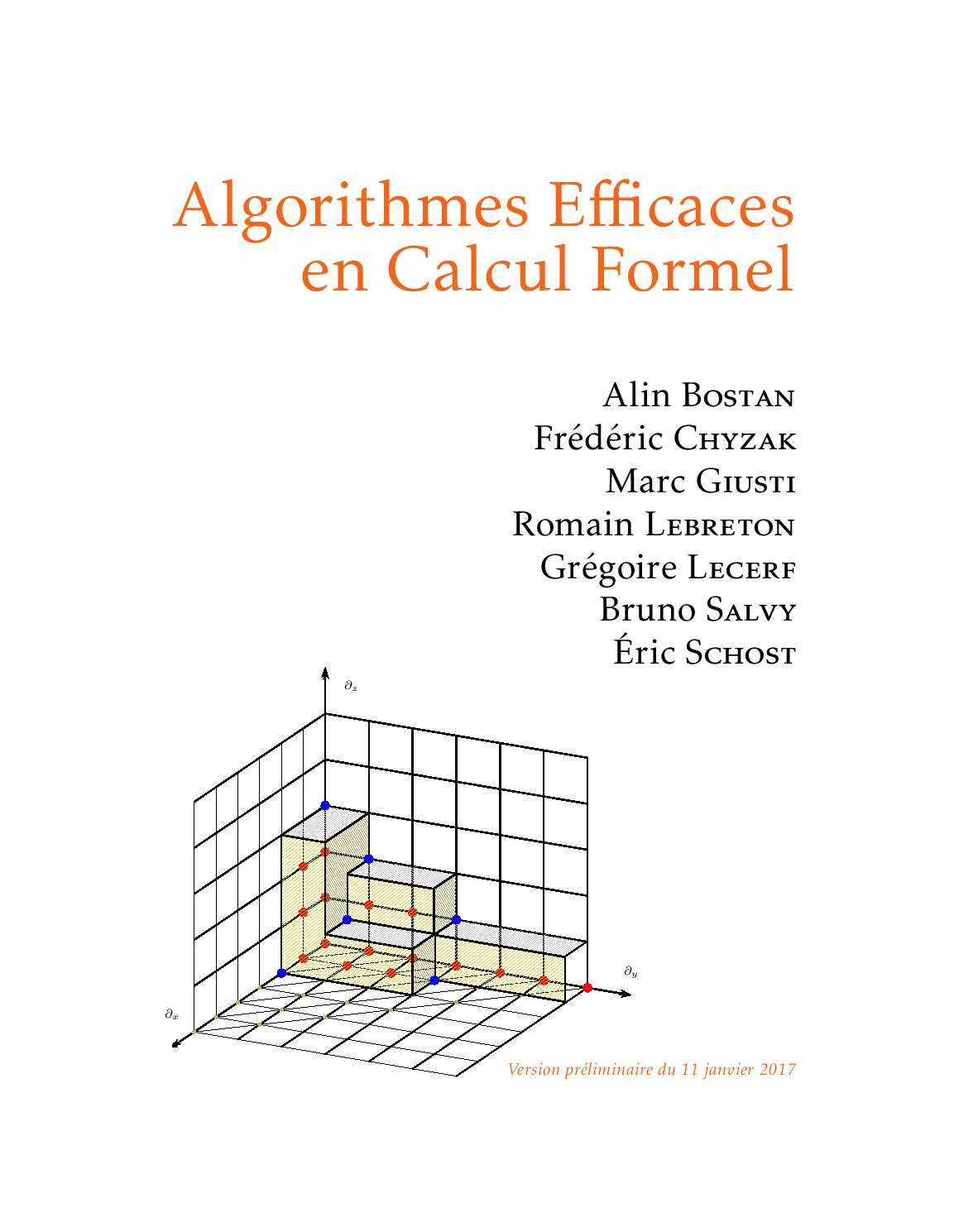In August 2017, we finally published a book version of the notes of the course on efficient algorithms for computer algebra we have been giving for more than 10 years. Click on the cover to reach a page where you can download it for free or order an inexpensive paper version.

## Recent WorkA lot of information concerning solutions of linear differential equations can be computed directly from the equation. It is therefore natural to consider these equations as a data-structure, from which mathematical properties can be computed. A variety of algorithms has thus been designed in recent years that do not aim at “solving”, but at computing with this representation. Many of these results are surveyed in this article.

B. Salvy, “Linear Differential Equations as a Data-Structure,” Foundations of Computational Mathematics, 2019.Definite integrals involving special functions and sequences can be dealt with automatically with a computer algebra technique called creative telescoping. Classical algorithms rely on the computation of an intermediate object called a certificate, whose size can be large and whose computation may be expensive. It turns out that for a large class of integrals, this part of the computation can be avoided, speeding up the whole process.

A. Bostan, F. Chyzak, P. Lairez, and B. Salvy, “Generalized Hermite Reduction, Creative Telescoping and Definite Integration of D-Finite Functions,” in ISSAC’18—Proceedings of the 2018 ACM International Symposium on Symbolic and Algebraic Computation, 2018, pp. 95–102.The probabilistic behaviour of many data-structures, like the series-parallel graphs in the picture, can be analysed very precisely, thanks to a set of high-level tools provided by Analytic Combinatorics, as described in the book by Flajolet and Sedgewick. In this framework, recursive combinatorial definitions lead to generating function equations from which efficient algorithms can be designed for enumeration, random generation and, to some extent, asymptotic analysis. With a focus on random generation, this tutorial given at STACS first covers the basics of Analytic Combinatorics and then describes the idea of Boltzmann sampling and its realisation. The tutorial addresses a broad TCS audience and no particular pre-knowledge on analytic combinatorics is expected.

B. Salvy, “Recursive Combinatorial Structures: Enumeration, Probabilistic Analysis and Random Generation,” in 35th Symposium on Theoretical Aspects of Computer Science (STACS 2018), Dagstuhl, Germany, 2018, vol. 96, pp. 1:1–1:5.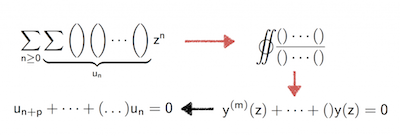Multiple binomial sums turn out to be diagonals of rational power series. This can be made effective, leading to an integral representation from which a linear differential equation for the generating function can be derived and from there finally a linear recurrence for the sum. This is a very efficient approach that avoids the computation of a large certificate.

A. Bostan, P. Lairez, and B. Salvy, “Multiple binomial sums,” Journal of Symbolic Computation, vol. 80, pp. 351–386, 2017.The three complex roots of the polynomial $$17x^3-9x^2-7x+8$$, depicted on the picture, lie on two different circles, at distance smaller than 0.000015 and thus indistinguishable on the picture. More generally, only pessimistic bounds are known for the distance between absolute values of roots of polynomials with integer coefficients. It turns out however, that in the case of real roots, tight bounds can be given.

Y. Bugeaud, A. Dujella, T. Pejkovic, and B. Salvy, “Absolute real root separation,” The American Mathematical Monthly, vol. 124, no. 10, pp. 930–936, Dec. 2017.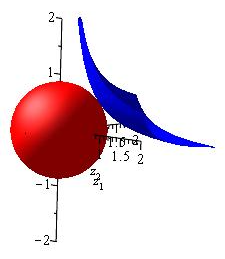Analytic combinatorics in several variables aims at finding the asymptotic behavior of sequences of diagonal coefficients of multivariate analytic functions. The starting point of the computation is the determination of the set of minimal critical points of the set of singularities. These are points where this set comes closest to the origin. In the case of rational functions with nonnegative coefficients, this can be achieved efficiently.

S. Melczer and B. Salvy, “Symbolic-Numeric Tools for Analytic Combinatorics in Several Variables,” in ISSAC’16: Proceedings of the 2016 ACM International Symposium on Symbolic and Algebraic Computation, New York, NY, USA, 2016, pp. 333–340.The picture on the right, from the cover of a book by Kenneth Chan on ‘‘Spacecraft collision probability’’, illustrates the quantity of debris orbiting around Earth and the importance of predicting collisions. We describe a method for the evaluation of the probability of such collisions using a closed form for the Laplace transform, an explicit recurrence for its Taylor coefficients and a technique to evaluate these in a numerically stable way. This method compares favorably to other existing techniques.

R. Serra, D. Arzelier, M. Joldes, J.-B. Lasserre, A. Rondepierre, and B. Salvy, “A New Method to Compute the Probability of Collision for Short-term Space Encounters,” in AIAA/AAS Astrodynamics Specialist Conference, 2014, pp. 1–7. R. Serra, D. Arzelier, M. Joldes, J.-B. Lasserre, A. Rondepierre, and B. Salvy, “Fast and accurate computation of orbital collision probability for short-term encounters,” Journal of Guidance, Control, and Dynamics, vol. 39, no. 5, pp. 1009–1021, 2016.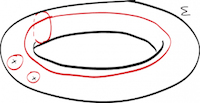Definite integrals of rational functions in several variables provide elementary representations for a large number of special functions, or combinatorial sequences through their generating functions. These integrals satisfy linear differential equations from which a lot of information about them can be extracted easily. The computation of these integrals is a classical topic, going back at least to Émile Picard, with recent progress based on creative telescoping.

A. Bostan, P. Lairez, and B. Salvy, “Creative telescoping for rational functions using the Griffiths-Dwork method,” in ISSAC ’13: Proceedings of the 38th International Symposium on Symbolic and Algebraic Computation, 2013, pp. 93–100. A. Bostan, L. Dumont, and B. Salvy, “Efficient Algorithms for Mixed Creative Telescoping,” in ISSAC’16—Proceedings of the 2016 ACM International Symposium on Symbolic and Algebraic Computation, 2016, pp. 127–134.Continued fractions provide a sequence of approximations to a number or a function in the same way as series expansions or infinite products do. For a large number of special functions, these continued fractions have coefficients given by an explicit rational function of the index. To a large extent, these formulas can be discovered and proved by computer algebra.

S. Maulat and B. Salvy, “Formulas for Continued Fractions: An Automated Guess and Prove Approach,” in ISSAC’15: Proceedings of the 2015 ACM International Symposium on Symbolic and Algebraic Computation, New York, NY, USA, 2015, pp. 275–282.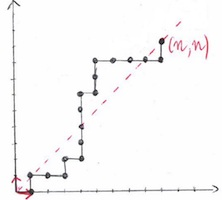Diagonals of multivariate rational functions encode the enumeration sequences of many combinatorial sequences. In the bivariate case, these diagonals are algebraic power series and an alternative representation is by their minimal polynomial. Yet another possibility is to use a linear differential equation they satisfy. Precise estimates of the sizes of these equations and of the complexity of obtaining them can be computed, showing that the differential equation is generally a better choice.

A. Bostan, L. Dumont, and B. Salvy, “Algebraic Diagonals and Walks,” in ISSAC’15: Proceedings of the 2015 ACM International Symposium on Symbolic and Algebraic Computation, New York, NY, USA, 2015, pp. 77–84. A. Bostan, L. Dumont, and B. Salvy, “Algebraic Diagonals and Walks: Algorithms, Bounds, Complexity,” Journal of Symbolic Computation, vol. 83, pp. 68–92, 2017.

The full collection of my recent (and not so recent) papers is here.